Courses

# Legendre Special Function (Part - 2) - Mathematical Methods of Physics, UGC - NET Physics Physics Notes | EduRev

## Physics for IIT JAM, UGC - NET, CSIR NET

Created by: Akhilesh Thakur

## Physics : Legendre Special Function (Part - 2) - Mathematical Methods of Physics, UGC - NET Physics Physics Notes | EduRev

The document Legendre Special Function (Part - 2) - Mathematical Methods of Physics, UGC - NET Physics Physics Notes | EduRev is a part of the Physics Course Physics for IIT JAM, UGC - NET, CSIR NET.
All you need of Physics at this link: Physics

RECURRENCE RELATIONS AND SPECIAL PROPERTIES

Recurrence Relations

The Legendre polynomial generating function provides a convenient way of deriving the recurrence relations and some special properties. If our generating function (Eq. (12.4)) is differentiated with respect to t , we obtain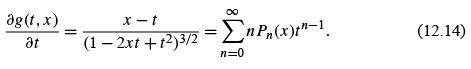By substituting Eq. (12.4) into this and rearranging terms, we have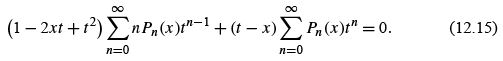The left-hand side is a power series in t . Since this power series vanishes for all values of t , the coefﬁcient of each power of t is equal to zero; that is, our power series is unique (Section 5.7). These coefﬁcients are found by separating the individual summations and using distinctive summation indices: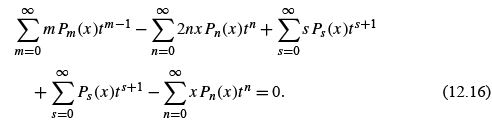Now, letting m = n + 1,s = n − 1, we ﬁnd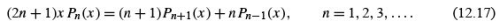.This is another three-term recurrence relation, similar to (but not identical with) the recurrence relation for Bessel functions. With this recurrence relation we may easily construct the higher Legendre polynomials. If we take n = 1 and insert the easily found values of P0 (x ) and P1 (x ) , we obtain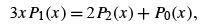(12.18)

or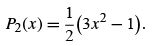(12.19)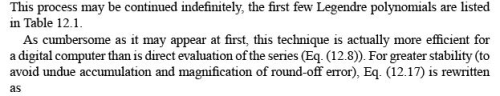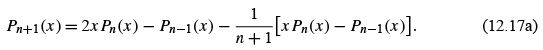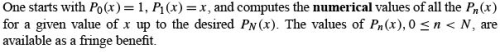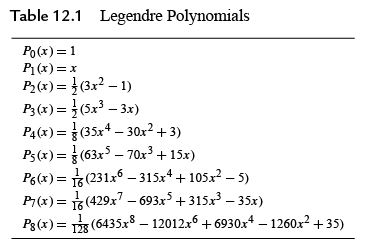Differential Equations

More information about the behavior of the Legendre polynomials can be obtained if we now differentiate Eq. (12.4) with respect to x . This gives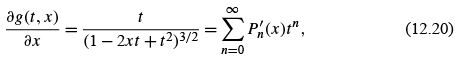or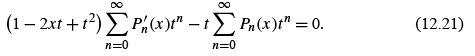As before, the coefﬁcient of each power of t is set equal to zero and we obtain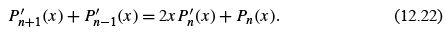A more useful relation may be found by differentiating Eq. (12.17) with respect to x and multiplying by 2. To this we add (2n + 1) times Eq. (12.22), canceling the Pn′ term. The result isFrom Eqs. (12.22) and (12.23) numerous additional equations may be developed,5 including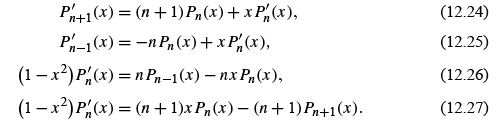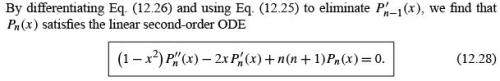The previous equations, Eqs. (12.22) to (12.27), are all ﬁrst-order ODEs, but with polynomials of two different indices. The price for having all indices alike is a second-order differential equation. Equation (12.28) is Legendre’s ODE. We now see that the polynomials Pn (x ) generated by the power series for (1 − 2xt + t 2 )−1/2 satisfy Legendre’s equation, which, of course, is why they are called Legendre polynomials.
In Eq. (12.28) differentiation is with respect to x(x = cos θ). Frequently, we encounter Legendre’s equation expressed in terms of differentiation with respect to θ :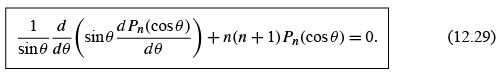Special Values

If we set x = 1, Eq. (12.4) becomes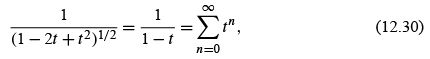using a binomial expansion or the geometric series, Example 5.1.1. But Eq. (12.4) for x = 1 deﬁnes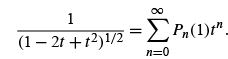Comparing the two series expansions (uniqueness of power series, Section 5.7), we have

Pn (1) = 1.                           (12.31)

If we let x =−1 in Eq. (12.4) and use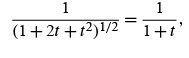this shows that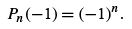(12.32)

For obtaining these results, we ﬁnd that the generating function is more convenient than the explicit series form, Eq. (12.8).
If we take x = 0 in Eq. (12.4), using the binomial expansion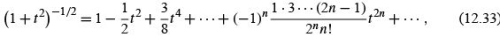we have6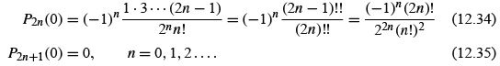These results also follow from Eq. (12.8) by inspection.

Parity

Some of these results are special cases of the parity property of the Legendre polynomials.

We refer once more to Eqs. (12.4) and (12.8). If we replace x by −x and t by −t ,the generating function is unchanged. Hence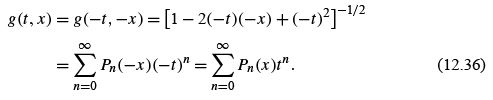Comparing these two series, we have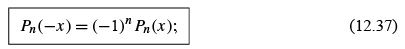that is, the polynomial functions are odd or even (with respect to x = 0,θ = π/2) according to whether the index n is odd or even. This is the parity,7 or reﬂection, property that plays such an important role in quantum mechanics. For central forces the index n is a measure of the orbital angular momentum, thus linking parity and orbital angular momentum.
This parity property is conﬁrmed by the series solution and for the special values tabulated in Table 12.1. It might also be noted that Eq. (12.37) may be predicted by inspection of Eq. (12.17), the recurrence relation. Speciﬁcally, if Pn−1 (x ) and xPn (x ) are even, then Pn+1 (x ) must be even.

Upper and Lower Bounds for Pn(cos θ)

Finally, in addition to these results, our generating function enables us to set an upper limit on |Pn (cos θ)|.Wehave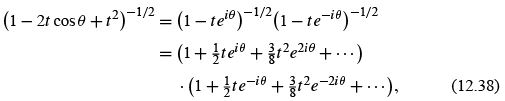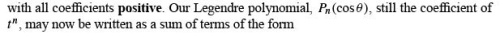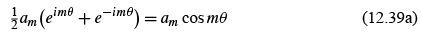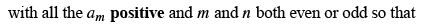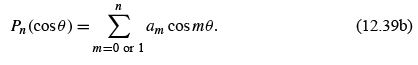This series, Eq. (12.39b), is clearly a maximum when θ = 0 and cos mθ = 1. But for x = cos θ = 1, Eq. (12.31) shows that P(1) = 1. Therefore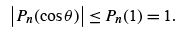(12.39c)

A fringe beneﬁt of Eq. (12.39b) is that it shows that our Legendre polynomial is a linear combination of cos mθ . This means that the Legendre polynomials form a complete set for any functions that may be expanded by a Fourier cosine series (Section 14.1) over the interval [0,π ].

• In this section various useful properties of the Legendre polynomials are derived from the generating function, Eq. (12.4).
• The explicit series representation, Eq. (12.8), offers an alternate and sometimes superior approach.

ORTHOGONALITY

Legendre’s ODE (12.28) may be written in the form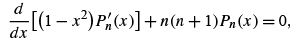(12.40)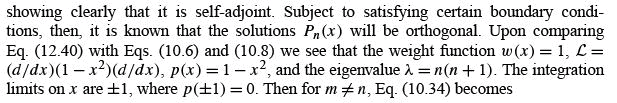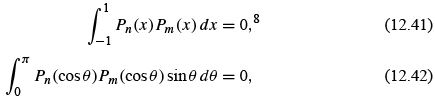showing that Pn (x ) and Pm (x ) are orthogonal for the interval [−1, 1]. This orthogonality may also be demonstrated by using Rodrigues’ deﬁnition of P(x ).We shall need to evaluate the integral (Eq. (12.41)) when n = m. Certainly it is no longer zero. From our generating function,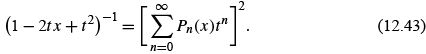Integrating from x =−1to x =+1, we have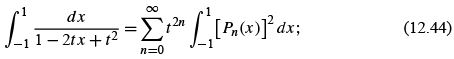the cross terms in the series vanish by means of Eq. (12.42). Using y = 1 − 2tx + t 2 , dy =−2td x , we obtain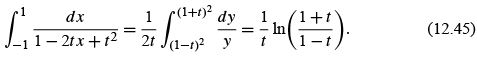Expanding this in a power series  gives us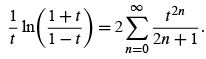(12.46)

Comparing power-series coefﬁcients of Eqs. (12.44) and (12.46), we must have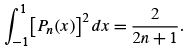(12.47)

Combining Eq. (12.42) with Eq. (12.47) we have the orthonormality condition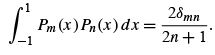(12.48)

We shall return to this result, when we construct the orthonormal spherical harmonics.

Expansion of Functions, Legendre Series

In addition to orthogonality, the Sturm–Liouville theory implies that the Legendre polynomials form a complete set. Let us assume, then, that the series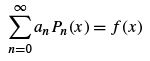(12.49)

converges in the mean (Section 10.4) in the interval [−1, 1]. This demands that f(x ) and f ′ (x ) be at least sectionally continuous in this interval. The coefﬁcients an are found by multiplying the series by Pm (x ) and integrating term by term. Using the orthogonality property expressed in Eqs. (12.42) and (12.48), we obtain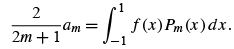(12.50)

We replace the variable of integration x by t and the index m by n. Then, substituting into Eq. (12.49), we have(12.51)

This expansion in a series of Legendre polynomials is usually referred to as a Legendre series. Its properties are quite similar to the more familiar Fourier series . In particular, we can use the orthogonality property (Eq. (12.48)) to show that the series is unique.
On a more abstract (and more powerful) level, Eq. (12.51) gives the representation of f(x ) in the vector space of Legendre polynomials .
From the viewpoint of integral transforms, Eq. (12.50) may be considered a ﬁnite Legendre transform of f(x ). Equation (12.51) is then the inverse transform. It may also be interpreted in terms of the projection operators of quantum theory. We may take Pm in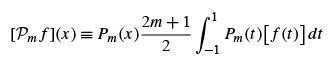as an (integral) operator, ready to operate on f(t ).(The f(t ) would go in the square bracket as a factor in the integrand.) Then, from Eq. (12.50),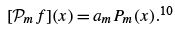The operator Pm projects out the mth component of the function f .
Equation (12.3), which leads directly to the generating function deﬁnition of Legendre polynomials, is a Legendre expansion of 1/r. This Legendre expansion of 1/r1 or 1/r12 . Going beyond a Coulomb ﬁeld, the 1 /r12 is often replaced by a potential V(|r− r2 |), and the solution of the problem is again effected by a Legendre expansion.

The Legendre series, Eq. (12.49), has been treated as a known function f(x ) that we arbitrarily chose to expand in a series of Legendre polynomials. Sometimes the origin and nature of the Legendre series are different. In the next examples we consider unknown functions we know can be represented by a Legendre series because of the differential equation the unknown functions satisfy. As before, the problem is to determine the unknown coefﬁcients in the series expansion. Here, however, the coefﬁcients are not found by Eq. (12.50). Rather, they are determined by demanding that the Legendre series match a known solution at a boundary. These are boundary value problems.

Offer running on EduRev: Apply code STAYHOME200 to get INR 200 off on our premium plan EduRev Infinity!

159 docs

,

,

,

,

,

,

,

,

,

,

,

,

,

,

,

,

,

,

,

,

,

,

,

,

;